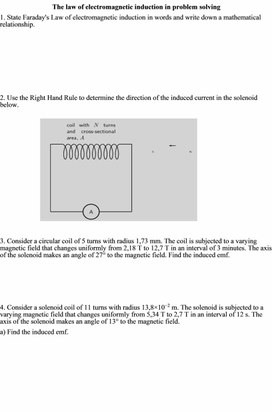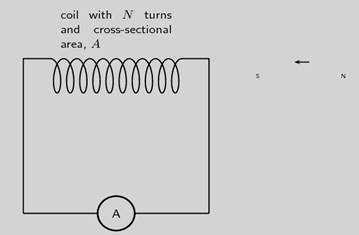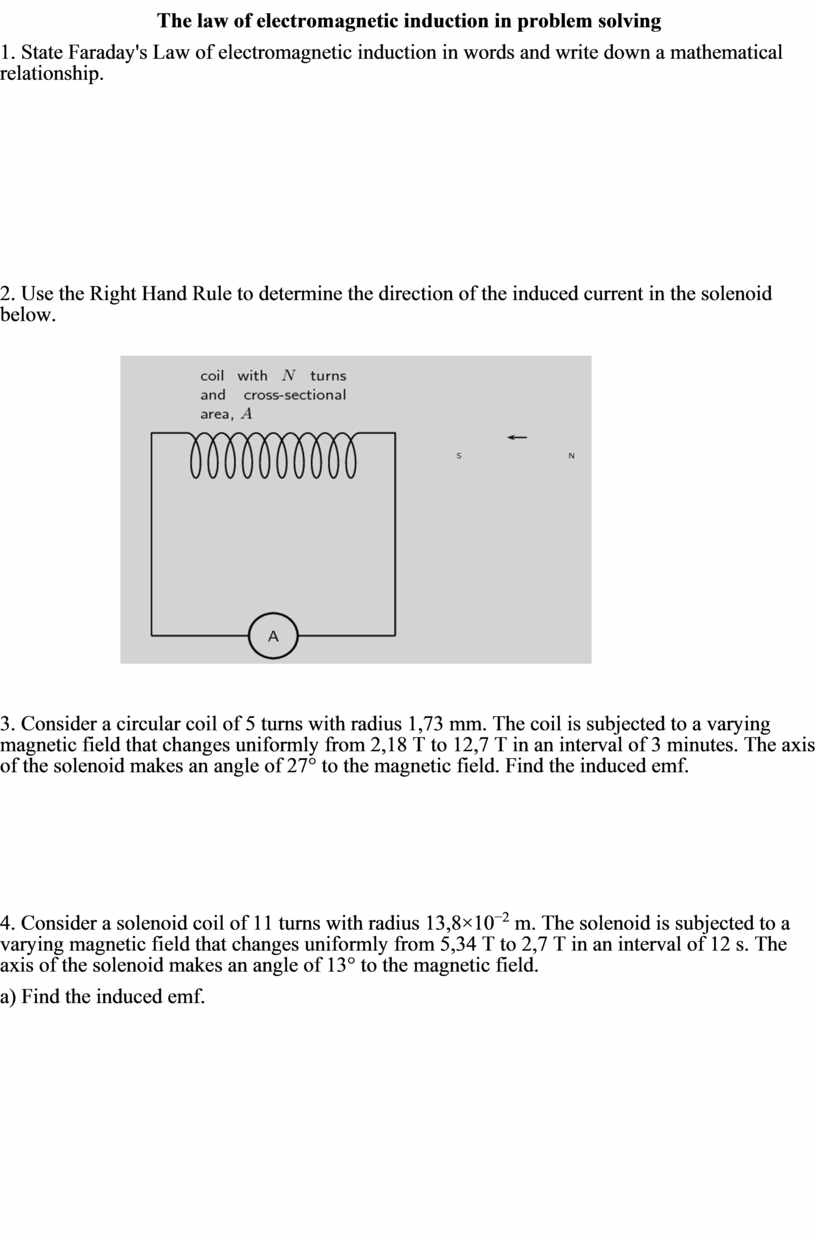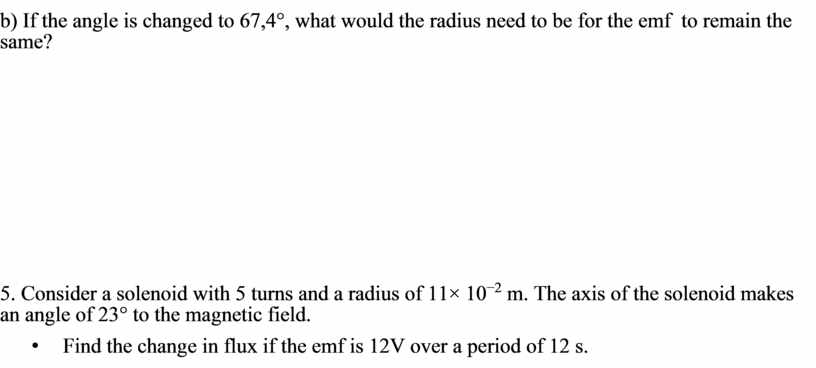1Lenz rule, phenomenon of self-inductance
Оценка 4.7

# 1Lenz rule, phenomenon of self-inductance

Оценка 4.7
docx
07.05.20201Lenz rule, phenomenon of self-inductance.docx

The law of electromagnetic induction in problem solving

1. State Faraday's Law of electromagnetic induction in words and write down a mathematical relationship.

2. Use the Right Hand Rule to determine the direction of the induced current in the solenoid below.3. Consider a circular coil of 5 turns with radius 1,73 mm. The coil is subjected to a varying magnetic field that changes uniformly from 2,18 T to 12,7 T in an interval of 3 minutes. The axis of the solenoid makes an angle of 27° to the magnetic field. Find the induced emf.

4. Consider a solenoid coil of 11 turns with radius 13,8×10−2 m. The solenoid is subjected to a varying magnetic field that changes uniformly from 5,34 T to 2,7 T in an interval of 12 s. The axis of the solenoid makes an angle of 13° to the magnetic field.

a) Find the induced emf.

b) If the angle is changed to 67,4°, what would the radius need to be for the emf  to remain the same?

5. Consider a solenoid with 5 turns and a radius of 11× 10−2 m. The axis of the solenoid makes an angle of 23° to the magnetic field.

Find the change in flux if the emf is 12V over a period of 12 s.

## The law of electromagnetic induction in problem solving 1## If the angle is changed to 67,4°, what would the radius need to be for the emf to remain the same? 5Скачать файл